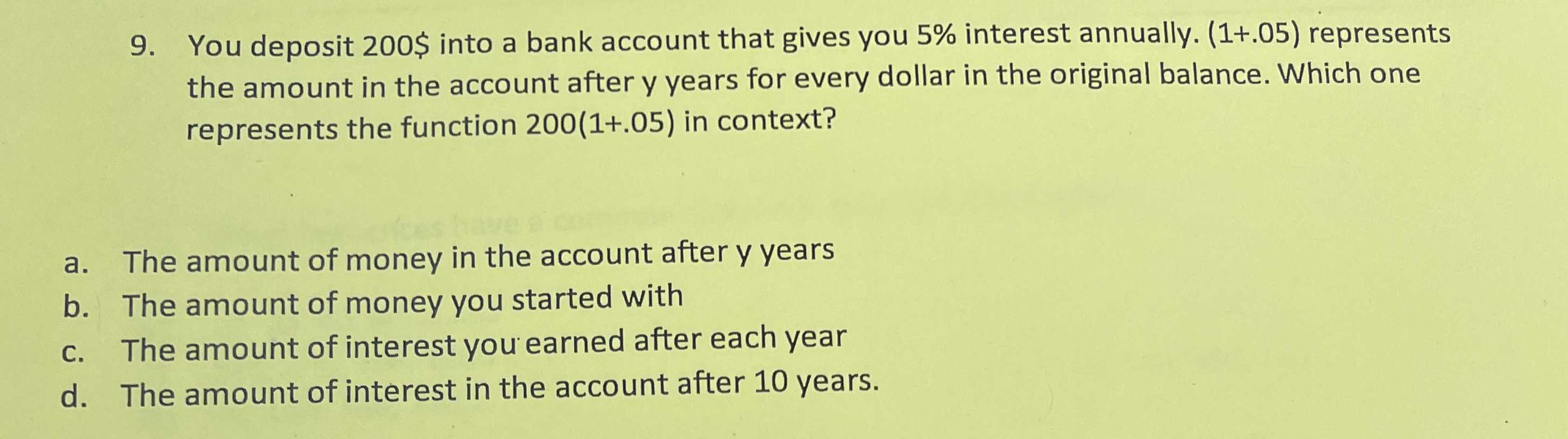### ¿Todavía tienes preguntas de matemáticas?

Pregunte a nuestros tutores expertos
Algebra
Pregunta9. You deposit $$200 \$$ into a bank account that gives you $$5 \%$$ interest annually. (1+.05) represents the amount in the account after y years for every dollar in the original balance. Which one represents the function $$200 ( 1 + .05 )$$ in context?

a. The amount of money in the account after y years

b. The amount of money you started with

c. The amount of interest you earned after each year

d. The amount of interest in the account after $$10$$ years.